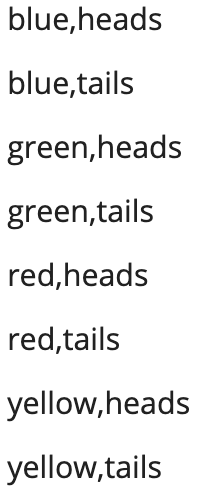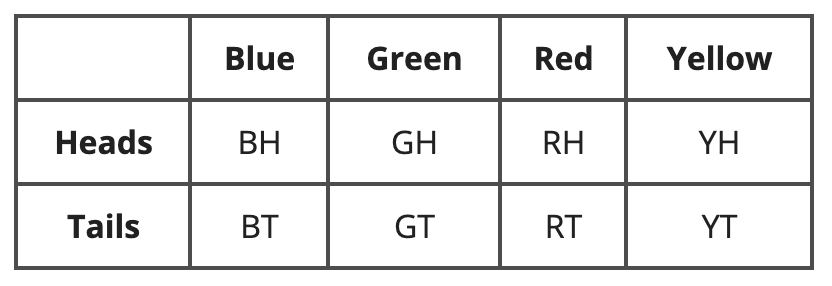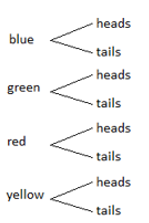# Probability

Students have their first encounter with probability, as they develop their understanding of probability through calculating theoretical probabilities and designing and running their own simulations.

## Unit Summary

In Unit 8, seventh-grade students finish the year with their first encounter with probability. They develop their understanding of probability through analyzing experiments, calculating theoretical probabilities, and designing and running their own simulations to model real-world situations (MP.4). Students encounter and use a variety of tools including spinners, dice, cards, coins, etc., and organizational tools such as organized lists, tables, and tree diagrams when they study compound probability (MP.5).

Students draw on and re-engage with concepts from ratios and proportions in order to fully understand probability as a ratio of desired outcomes to total outcomes. They also use proportional relationships to estimate long-run frequencies based on probabilities of experiments.

In high school, students will further explore probability, distinguishing between independent events and conditional events and developing rules to calculate probabilities of these compound events.

Pacing: 11 instructional days (9 lessons, 1 flex day, 1 assessment day)

For guidance on adjusting the pacing for the 2021-2022 school year, see our 7th Grade Scope and Sequence Recommended Adjustments.• Expanded Assessment Package
• Problem Sets for Each Lesson
• Student Handout Editor
• Vocabulary Package

## Assessment

This assessment accompanies Unit 8 and should be given on the suggested assessment day or after completing the unit.

## Unit Prep

### Intellectual Prep

?

#### Internalization of Standards via the Post-Unit Assessment

• Take the Post-Unit Assessment. Annotate for:
• Standards that each question aligns to
• Strategies and representations used in daily lessons
• Relationship to Essential Understandings of unit
• Lesson(s) that Assessment points to

#### Internalization of Trajectory of Unit

• Read and annotate the Unit Summary.
• Notice the progression of concepts through the unit using the Lesson Map.
• Essential Understandings
• Connection to Post-Unit Assessment questions
• Identify key opportunities to engage students in academic discourse. Read through our Teacher Tool on Academic Discourse and refer back to it throughout the unit.

#### Unit-Specific Intellectual Prep

 Model Example Organized list The sample space for a spinner with 4 equal sections labeled yellow, blue, green, and red, and a fair coin with sides heads and tailsTable The sample space for a spinner with 4 equal sections labeled yellow, blue, green, and red, and a fair coin with sides heads and tailsTree diagram The sample space for a spinner with 4 equal sections labeled yellow, blue, green, and red, and a fair coin with sides heads and tails### Essential Understandings

?

• The probability of an event or combination of events occurring is determined by the number of desired or favorable outcomes divided by the total number of outcomes possible. This value ranges from 0, where the event is impossible, to 1, where the event is certain to occur, with various levels of likelihood in between.
• Experimental or theoretical probabilities can be used to estimate or predict long-run frequencies.
• Real-world situations can be simulated using various probability models in order to test hypotheses or make predictions based on data.

### Materials

?

• Dice (2 per student)
• Counters (11 per small group)
• Optional: Standard deck of playing cards (1 per small group)
• Brown bag (2 per pair of students)
• Cubes (1 set per small group) — 15 with 5 of one color and 10 of different color(s)
• Plastic cups (non-see-through ) (3 per small group)
• Plastic balls (like ping-pong) (3 per small group)
• Coins (1 per student)
• The Horse Race Board (1 per small group) — Found on page S-3 of Analyzing Games of Chance
• Race Results Sheet (1 per small group) — Found on page S-5 of Analyzing Games of Chance
• Optional: Spinners (1 per small group)

### Vocabulary

?

tree diagram

experimental probability

likelihood

probability

sample space

outcome

theoretical probability

simulation

simple event

compound event

To see all the vocabulary for this course, view our 7th Grade Vocabulary Glossary.

# 1

7.SP.C.5

Understand the probability of an event happening is a number between 0 and 1, ranging from impossible to certain.

# 2

7.SP.C.6

7.SP.C.7

Define probability and sample space. Estimate probabilities from experimental data.

# 3

7.SP.C.7.A

7.SP.C.7.B

Determine the probability of events.

# 4

7.SP.C.6

Use probability to predict long-run frequencies.

# 5

7.SP.C.7

Design and conduct simulations to model real-world situations.

# 6

7.SP.C.8

7.SP.C.8.C

Conduct simulations with multiple events to determine probabilities.

# 7

7.SP.C.8.B

List the sample space for compound events using organized lists, tables, or tree diagrams.

# 8

7.SP.C.8

Determine the probability of compound events.

# 9

7.SP.C.8.C

Design and conduct simulations to model real-world situations for compound events.

## Common Core Standards

Key: Major Cluster Supporting Cluster Additional Cluster

### Core Standards

?

##### Statistics and Probability
• 7.SP.C.5 — Understand that the probability of a chance event is a number between 0 and 1 that expresses the likelihood of the event occurring. Larger numbers indicate greater likelihood. A probability near 0 indicates an unlikely event, a probability around 1/2 indicates an event that is neither unlikely nor likely, and a probability near 1 indicates a likely event.

• 7.SP.C.6 — Approximate the probability of a chance event by collecting data on the chance process that produces it and observing its long-run relative frequency, and predict the approximate relative frequency given the probability. For example, when rolling a number cube 600 times, predict that a 3 or 6 would be rolled roughly 200 times, but probably not exactly 200 times.

• 7.SP.C.7 — Develop a probability model and use it to find probabilities of events. Compare probabilities from a model to observed frequencies; if the agreement is not good, explain possible sources of the discrepancy.

• 7.SP.C.7.A — Develop a uniform probability model by assigning equal probability to all outcomes, and use the model to determine probabilities of events. For example, if a student is selected at random from a class, find the probability that Jane will be selected and the probability that a girl will be selected.

• 7.SP.C.7.B — Develop a probability model (which may not be uniform) by observing frequencies in data generated from a chance process. For example, find the approximate probability that a spinning penny will land heads up or that a tossed paper cup will land open-end down. Do the outcomes for the spinning penny appear to be equally likely based on the observed frequencies?

• 7.SP.C.8 — Find probabilities of compound events using organized lists, tables, tree diagrams, and simulation.

• 7.SP.C.8.A — Understand that, just as with simple events, the probability of a compound event is the fraction of outcomes in the sample space for which the compound event occurs.

• 7.SP.C.8.B — Represent sample spaces for compound events using methods such as organized lists, tables and tree diagrams. For an event described in everyday language (e.g., "rolling double sixes"), identify the outcomes in the sample space which compose the event.

• 7.SP.C.8.C — Design and use a simulation to generate frequencies for compound events. For example, use random digits as a simulation tool to approximate the answer to the question: If 40% of donors have type A blood, what is the probability that it will take at least 4 donors to find one with type A blood?

?

• 5.NF.A.2

• 7.RP.A.2

• 7.RP.A.3

• 7.SP.A.1

?

• HSS-CP.A.1

• HSS-CP.A.2

• HSS-CP.A.3

• HSS-CP.A.4

• HSS-CP.A.5

• HSS-CP.B.6

• HSS-CP.B.7

• HSS-CP.B.8

• HSS-CP.B.9

• 8.SP.A.4

• HSS-MD.A.3

• HSS-MD.A.4

### Standards for Mathematical Practice

• CCSS.MATH.PRACTICE.MP1 — Make sense of problems and persevere in solving them.

• CCSS.MATH.PRACTICE.MP2 — Reason abstractly and quantitatively.

• CCSS.MATH.PRACTICE.MP3 — Construct viable arguments and critique the reasoning of others.

• CCSS.MATH.PRACTICE.MP4 — Model with mathematics.

• CCSS.MATH.PRACTICE.MP5 — Use appropriate tools strategically.

• CCSS.MATH.PRACTICE.MP6 — Attend to precision.

• CCSS.MATH.PRACTICE.MP7 — Look for and make use of structure.

• CCSS.MATH.PRACTICE.MP8 — Look for and express regularity in repeated reasoning.